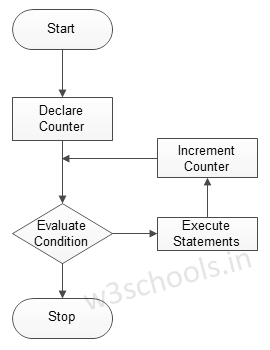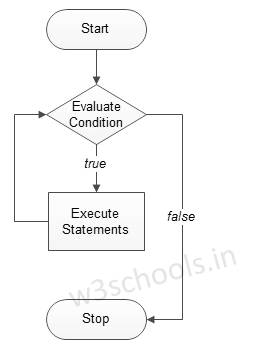##### Python Programming Tutorial Index

In programming, loops are a sequence of instructions that does a specific set of instructions or tasks based on some conditions and continue the tasks until it reaches certain conditions.

It is seen that in programming, sometimes we need to write a set of instructions repeatedly - which is a tedious task, and the processing also takes time. So in programming, we use iteration technique to repeat the same or similar type of tasks based on the specified condition.

Statements are executed sequentially, but there sometimes occur such cases where programmers need to execute a block of code several times. The control structures of programming languages allow us to execute a statement or block of statements repeatedly.

## Types of Loops in Python

Python provides three types of looping techniques:

Python Loops

Loop Description
for Loop This is traditionally used when programmers had a piece of code and wanted to repeat that 'n' number of times.
while Loop The loop gets repeated until the specific Boolean condition is met.
Nested Loops Programmers can use one loop inside another; i.e., they can use for loop inside while or vice - versa or for loop inside for loop or while inside while.

## for Loop

The graphical representation of the logic behind for looping is shown below:

Figure - for loop Flowchart:Syntax:
```for iterating_var in sequence:
Example 01:
``````for x in range (0,3) :
print ('Loop execution %d' % (x))``````
Output:
```Loop execution 0
Loop execution 1
Loop execution 2```
Example 02:
``````for letter in 'TutorialsCloud':
print ('Current letter is:', letter)
``````
Output:
```Current letter is : T
Current letter is : u
Current letter is : t
Current letter is : o
Current letter is : r
Current letter is : i
Current letter is : a
Current letter is : l
Current letter is : s
Current letter is : C
Current letter is : l
Current letter is : o
Current letter is : u
Current letter is : d```

## while Loop

The graphical representation of the logic behind while looping is shown below:

Figure - while loop Flowchart:Syntax:
```while expression:
Example:
``````#initialize count variable to 1
count =1

while count < 6 :
print (count)
count+=1
#the above line means count = count + 1``````
Output:
```1
2
3
4
5```

## Nested Loops

Syntax:
```for iterating_var in sequence:
for iterating_var in sequence:
Example:
``````for g in range(1, 6):
for k in range(1, 3):
print ("%d * %d = %d" % ( g, k, g*k))``````
Output:
```1 * 1 = 1
1 * 2 = 2
2 * 1 = 2
2 * 2 = 4
3 * 1 = 3
3 * 2 = 6
4 * 1 = 4
4 * 2 = 8
5 * 1 = 5
5 * 2 = 10```

## Loop Control Statements

These statements are used to change execution from its normal sequence.

Python supports three types of loop control statements:

Python Loop Control Statements

Control Statements Description
Break statement It is used to exit a while loop or a for a loop. It terminates the looping & transfers execution to the statement next to the loop.
Continue statement It causes the looping to skip the rest part of its body & start re-testing its condition.
Pass statement It is used in Python to when a statement is required syntactically, and the programmer does not want to execute any code block or command.

### Break statement

Syntax:
`break`
Example:
``````count = 0

while count <= 100:
print (count)
count += 1
if count >= 3:
break``````
Output:
```0
1
2```

### Continue statement

Syntax:
`continue`
Example:
``````for x in range(10):
#check whether x is even
if x % 2 == 0:
continue
print (x)``````
Output:
```1
3
5
7
9```

### Pass Statement

Syntax:
`pass`
Example:
``````for letter in 'TutorialsCloud':
if letter == 'C':
pass
print ('Pass block')
print ('Current letter is:', letter)

``````
Output:
```Current letter is : T
Current letter is : u
Current letter is : t
Current letter is : o
Current letter is : r
Current letter is : i
Current letter is : a
Current letter is : l
Current letter is : s
Pass block
Current letter is : C
Current letter is : l
Current letter is : o
Current letter is : u
Current letter is : d```